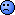## Help for ANOVA from Package replicateBE [🇷 for BE/BA]

Dear friends,

By studying the replicateBE package documentation https://github.com/Helmut01/replicateBE#readme I was able to verify that the method.A and method.B functions (package replicateBE) are derived from the functions lm and lme (packages stats and nlme).

If possible I would like to reproduce the same ANOVA tables that are displayed in the output of the methods.A and method.B using only the lm and lme functions as indicated in the instructions. Unfortunately I am not reaching my goal of obtain the same results as expected. The hipotetical data set that I working is from replicated 2x4 study and the ANOVA results to be different (GL, p-values, etc...). What am I doing wrong? Why can't I generate the same ANOVA Table? The my results to be bellow:

Somebody can help me?

I Would like publish at forum my data set that generates this results however I didn't see option for upload files excel (just figures). Is it possible publish excel files? If yes, How?

R Code 1.0
method.A with IPC_Drug.Cmax Data Set

method.A(path.in = path, path.out = path, file = paste0(fn, ".", "Cmax"),  ext = "csv", na = "NA", sep = sep, dec = dec, ola = TRUE, print = TRUE, verbose = TRUE, ask = TRUE, plot.bxp = TRUE)

ANOVA Outputs for R Code 1.0:

Data set IPC_Drug.Cmax: Method A by lm() ──────────────────────────────────────── Type III Analysis of Variance Table Response: log(PK)                            Df    Sum Sq       Mean Sq    F value      Pr(>F) sequence                   1      1.3253      1.32531    0.27224    0.604233 period                     3      3.5824      1.19413    2.19909    0.090673 treatment                  1      2.7923      2.79228    5.14222    0.024818 sequence:subject           48   233.6721      4.86817    8.96514    < 2e-16 Residuals                 146    79.2796      0.54301                  treatment T – R:   Estimate Std. Error    t value   Pr(>|t|) -0.2380370  0.1049710 -2.2676500  0.0248184 146 Degrees of Freedom 

R Code 1.1
Obtain ANOVA by lm() function with DS_Cmax Data Set

library(stats) Fit_A <- lm(log(Cmax) ~ Sequence + Subject%in%Sequence + Period + Treatment, data=DS_Cmax) anova(Fit_A) 

ANOVA Outputs for R Code 1.1:

Analysis of Variance Table Response: log(Cmax)                         Df       Sum Sq           Mean Sq           F value       Pr(>F) Sequence                 1       1.32531015       1.325310155       0.83233     0.36273 Period                   1       0.31093557       0.310935573       0.19528     0.65905 Treatment                1      3.41095080        3.410950796       2.14218     0.14492 Sequence:Subject         2      6.70138392        3.350691961       2.10433     0.12470 Residuals                194   308.90305612       1.592283794 

R Code 2.0
method.B with IPC_Drug.Cmax Data Set

method.B(path.in = path, path.out = path, file = paste0(fn, ".", "Cmax"), ext = "csv", na = "NA", sep = sep, dec = dec, ola = TRUE,  print = TRUE, verbose = TRUE, ask = TRUE, plot.bxp = TRUE)

ANOVA Outps for utR Code 2.0:

Data set IPC_Drug.Cmax: Method B (option = 2) by lme() ────────────────────────────────────────────────────── Response: log(PK)                 numDF       denDF       F-value              p-value (Intercept)      1           146      763.258972853        <.0001 sequence         1            48      0.272239993          0.6042 period           3           146      2.199092956          0.0907 treatment        1           146      5.142222444          0.0248 treatment T – R:     Value Std.Error   t-value   p-value -0.238040  0.104970 -2.267600  0.024818 146 Degrees of Freedom (equivalent to SAS’ DDFM=CONTAIN) 

R Code 2.1
Obtain ANOVA by lme() function with DS_Cmax Data Set

library(nlme) Fit_B_opt2 <- lme (log(Cmax)~ Sequence +  Period + Treatment, random = ~1|Subject, data=DS_Cmax) anova(Fit_B_opt2)

ANOVA Outputs for R Code 2.1:

               numDF       denDF       F-value            p-value (Intercept)     1           148       763.258972846       <.0001 Sequence        1            48         0.272239993       0.6042 Period          1           148         0.561664145       0.4548 Treatment       1           148         6.161433208       0.0142Ing. Helmut Schütz